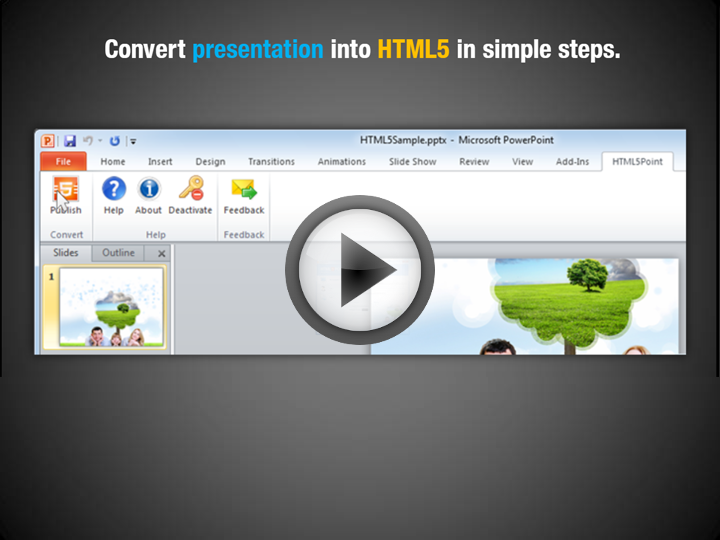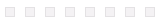﻿ HTML5_DEMO00:00

/ 8

`W`
`o`
`r`
`l`
`d`
`’`
`s`
`F`
`i`
`r`
`s`
`t`
`P`
`o`
`w`
`e`
`r`
`P`
`o`
`i`
`n`
`t`
`t`
`o`
`H`
`T`
`M`
`L`
`5`
`C`
`o`
`n`
`v`
`e`
`r`
`t`
`e`
`r`
`This slideshow is created with HTML5Point software`
`Convert `
`Presentation`
` into `
`HTML5`
`in simple steps.`
`Convert `
`presentation`
` into `
`HTML5`
` in simple steps.`
`Supports all `
`HTML5`
`supported devices.`
`iPhone`
`iPad`
`Android Devices`
`Retains Special Effects Like..`

``
`L`
`e`
`t`
`t`
`e`
`r`
`B`
`y`
`L`
`e`
`t`
`t`
`e`
`r`

``
`Word `
`By `
`Word`
`Animations And Transitions`
`Multimedia Support`
``
`Embedded Videos`
``
`Animated Gifs`

`A`
`n`
`d`
`s`
`u`
`p`
`p`
`o`
`r`
`t`
`s`
`a`
`l`
`l`
`m`
`a`
`j`
`o`
`r`
`W`
`e`
`b`
`B`
`r`
`o`
`w`
`s`
`e`
`r`
`s`

`C`
`o`
`n`
`v`
`e`
`r`
`t`
`e`
`d`
`H`
`T`
`M`
`L`
`5`
`o`
`u`
`t`
`p`
`u`
`t`
`s`
`u`
`p`
`p`
`o`
`r`
`t`
`s`
`Apple`
`Android `
`Windows `
`Linux `
`Get our free version and discover just how easy it is to use!`
`POINT`
`HTML`
`Download Now`0